Over a million developers have joined DZone.{{announcement.body}}
{{announcement.title}}

nearForm Data Science: Exploration of Recurrent Units in RNN

DZone 's Guide to

nearForm Data Science: Exploration of Recurrent Units in RNN

An exploration of recent developments of recurrent units in recurrent neural networks (RNN) and their effect on contextual understanding in text.

· AI Zone ·
Free Resource

Comment (0)

Save
{{ articles.views | formatCount}} Views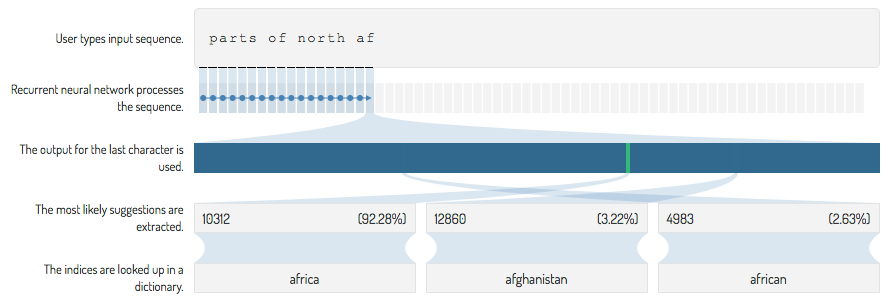Autocomplete: An example application showing how a simple recurrent neural network uses past information and understands the next word should be a region.

Introduction

Recent advances in handwriting recognition, speech recognition, and machine translation have with only a few exceptions (such as here and here) been based on recurrent neural networks.

Neural Networks

Recurrent neural networks are, funnily enough, a type of neural network. Neural networks have been around since at least 1975 but have over the recent years had a comeback and have become very popular. This is likely due to the advances in General Purpose GPU (GPGPU) programming, which provides the computational resources to train them and larger datasets that provide enough data to train large networks.

If you are not familiar with neural networks, it is recommended that you become at least a bit familiar. Today, there are many sources to learn from. The Neural Networks and Deep Learning book by Michael Nielsen is quite easy to get started with — Chapter 2 should give most of the required background. If you are more curious, the Deep Learning book by Goodfellow et al. is much more extensive; Chapter 5 should be a good start.

To give a short introduction, vanilla neural networks are essentially composed of two things: sums and non-linear function, like the sigmoid function. In matrix notation this can be written as: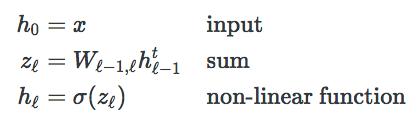...where x is the output.

In this article, the output is in terms of probabilities. To turn something into probabilities the Softmax function can be used.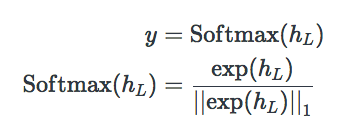Memorization Problem

The examples mentioned earlier may use additional techniques such as attention mechanisms to work with an unknown alignment between the source and the target sequence.

However, the foundation for these networks is still the recurrent neural network. Likewise, a common challenge for many of these applications is to get the network to memorize past content from the input sequences and use this for contextual understanding later in the sequence.

This memorization problem is what is explored in this article. To this end, this article doesn't go into the details of how to deal with an unknown alignment but rather focuses on problems where the alignment is known and explores the memorization issue for those problems. This is heavily inspired by the recent article on Nested LSTMs, which are also discussed in this article.

Recurrent Units

Recurrent neural networks (RNNs) are well-known and are thoroughly explained in literature. To keep it short, recurrent neural networks let you model a sequence of vectors. RNNs do this by iterating over the sequence, where each layer uses the output from the same layer in the previous "time" iteration, combined with the output from the previous layer in the same "time" iteration.

In theory, this type of network allows it in each iteration to know about every part of the sequence that came before.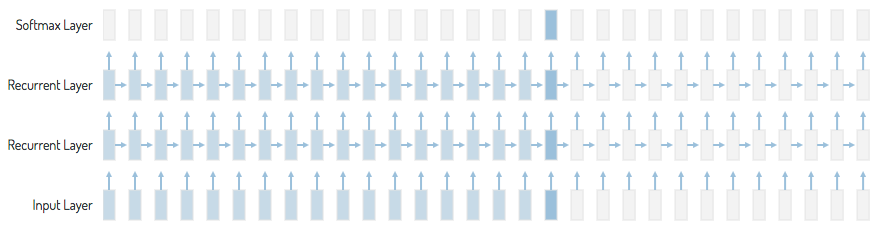Recurrent neural network, as used in an autocomplete example. This shows how the network, in theory, knows about every part of the sequence that came before.

Given an input sequence: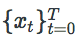Such a model can be expressed using the following set of equations: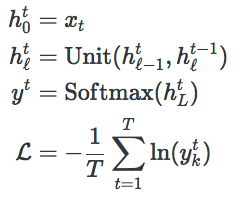Note how the output from the previous iteration and the output from the previous layer in the same iteration are combined is abstracted away.

For a vanilla recurrent neural network, the recurrent unit: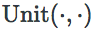Is: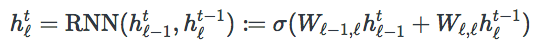Deep neural networks can suffer from a vanishing gradient problem where the gradient used in optimization becomes minuscule. This is because the δ used in backpropagation ends up being multiplicatively depending on the δ of the next layer.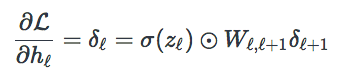This problem can be mitigated through careful initialization of the weights: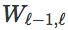By choosing an activation function σ such as the Rectified Linear Unit (ReLU), or adding residual connections.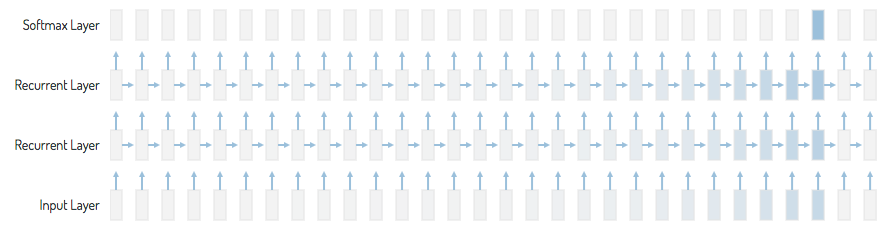Vanishing gradient: Where the contribution from the earlier steps becomes insignificant.

In classic recurrent neural networks, this problem becomes much worse due to the time dependencies, as the time dependencies essentially unfold into a potentially infinite deep neural network.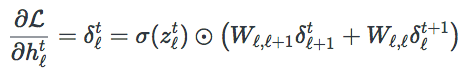An intuitive way of viewing this problem is that the vanilla recurrent network forces an update of the state: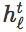This forced update is what courses the vanishing gradient problem. This forced update is also insufficient as irrelevant input data, such as skip words, blur out important information from previous iterations.

Long Short-Term Memory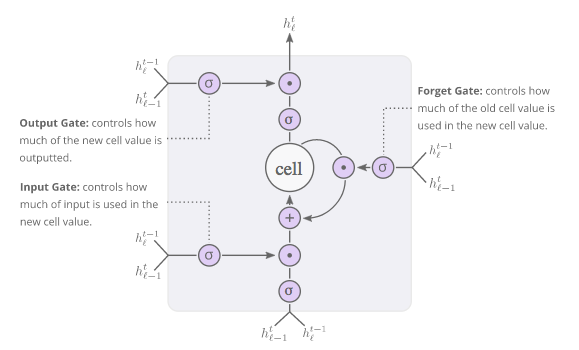LSTM: (Long Short-Term Memory): Allows for long-term memorization by gating its update, thereby solving the vanishing gradient problem.

The Long Short-Term Memory (LSTM) unit replaces the simple \mathrm{RNN}RNN unit from earlier. Each LSTM unit contains a single memory scalar that can be protected or written to, depending on the input and forget gate. This structure has shown to be very powerful in solving complex sequential problems. LSTM is well-known and thoroughly explained in the literature and therefore not discussed here. However, as it plays a critical part in the Nested LSTM unit, that is discussed later. Its equations are mentioned here.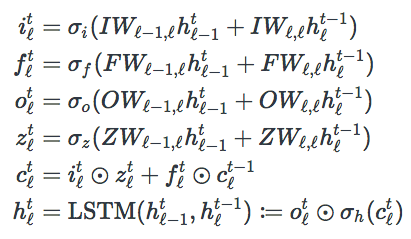The gate activation functions: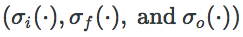Are usually the Sigmoid activation function, while: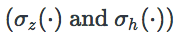Are usually tanh().

Nested LSTM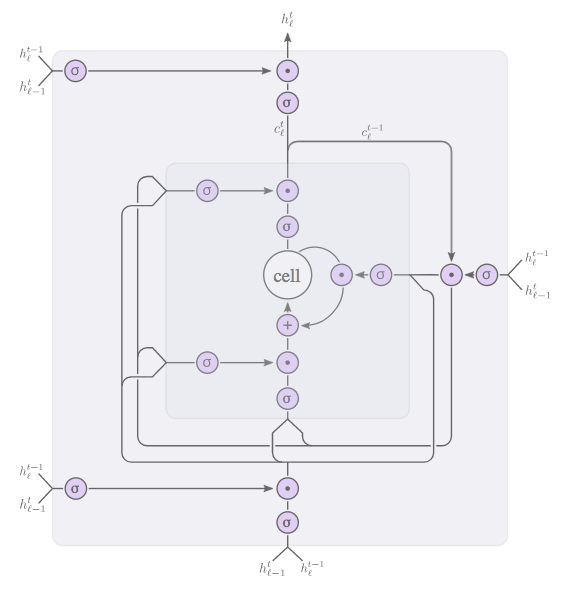Nested LSTM: Makes the cell update depend on another LSTM unit. Supposedly, this allows more long-term memory compared to stacking LSTM layers.

Even though the LSTM unit and GRU solves the vanishing gradient problem on a theoretical level, long-term memorization continues to be a challenge in recurrent neural networks.

There are alternatives to LSTM, most popular is the Gated Recurrent Unit (GRU). However, the GRU doesn’t necessarily give better long-term context, particularly as it solves the vanishing gradient problem without using any internal memory.

The Nested LSTM unit attempts to solve the long-term memorization from a more practical point of view. Where the classic LSTM unit solves the vanishing gradient problem by adding internal memory, and the GRU attempts to be a faster solution than LSTM by using no internal memory, the Nested LSTM goes in the opposite direction of GRU, as it adds additional memory to the unit.

The idea here is that adding additional memory to the unit allows for more long-term memorization.

The additional memory is integrated by changing how the cell value: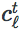Is updated. Instead of defining the cell value update as: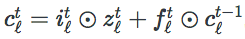It uses another LSTM unit: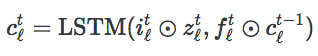Note that the variables defined in LSTM(,) are different from those defined below. The end result is that an NLSTM(,) unit has two memory states.

The complete set of equations then becomes: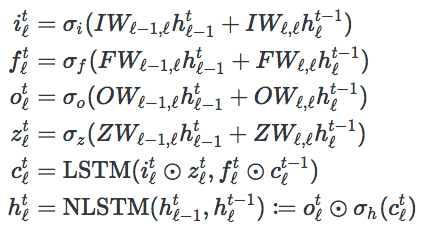Like in vanilla LSTM, the gate activation functions: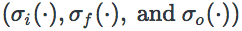Are usually the Sigmoid activation function. However, only the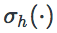Is set to tanh(), while: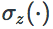Is just the identity function; otherwise, two non-linear activation functions would be applied on the same scalar without any change, except for the multiplication by the input gate. The activation functions for LSTM(,) remains the same.

The abstraction, of how to combine the input with the cell value, allows a lot of flexibility. Using this abstraction, it is not only possible to add one extra internal memory state but the internal LSTM(,) unit can recursively be replaced as many internal NLSTM(,) units as one would wish, thereby adding even more internal memory.

From a theoretical view, whether or not the Nested LSTM unit improves long context is not really clear. The LSTM unit theoretically solves the vanishing gradient problem and a network of LSTM units is Turing complete. In theory, an LSTM unit should be sufficient for solving problems that require long-term memorization.

That being said, it is often very difficult to train LSTM and GRU based recurrent neural networks. These difficulties often come down to the curvature of the loss function and it is possible that the Nested LSTM improves this curvature and therefore is easier to optimize.

Comparing Recurrent Units

Comparing the different Recurrent Units is not a trivial task. Different problems require different contextual understanding and therefore require different memorization.

A good problem for analyzing the contextual understanding should have a humanly interpretive output and depend both on long and short-term memorization.

To this end, the autocomplete problem is used. Each character is mapped to a target that represents the entire word. To make it extra difficult, the space leading up to the word should also map to that word. The text is from the full text8 dataset, where each observation consists of maximum 200 characters and is ensured to not contain partial words. 90% of the observations are used for training, 5% for validation, and 5% for testing.

The input vocabulary is a-z, space, and a padding symbol. The output vocabulary consists of the 2^{14}= 16384214=16384 most frequent words, and two additional symbols: one for padding and one for unknown words. The network is not penalized for predicting padding and unknown words wrong.

The GRU and LSTM models each have two layers of 600 units. Similarly, the Nested LSTM model has one layer of 600 units but with 2 internal memory states. Additionally, each model has an input embedding layer and a final dense layer to match the vocabulary size.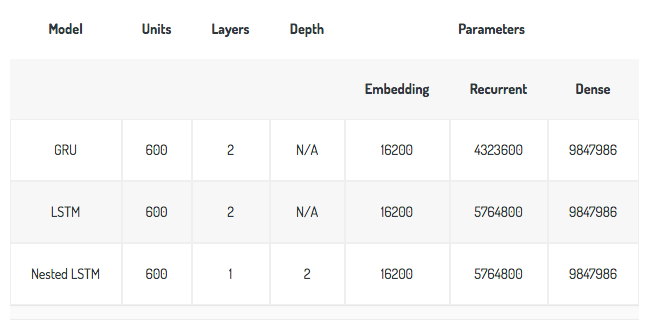Model configurations: Shows the number of layers, units, and parameters for each model.

There are 508,583 sequences in the training dataset and a batch size of 64 observations is used. A single iteration of the entire dataset then corresponds to 7,946 epochs, which is enough to train the network; therefore, the models only trained for 7,946 epochs. For training, Adam optimization is used with default parameters.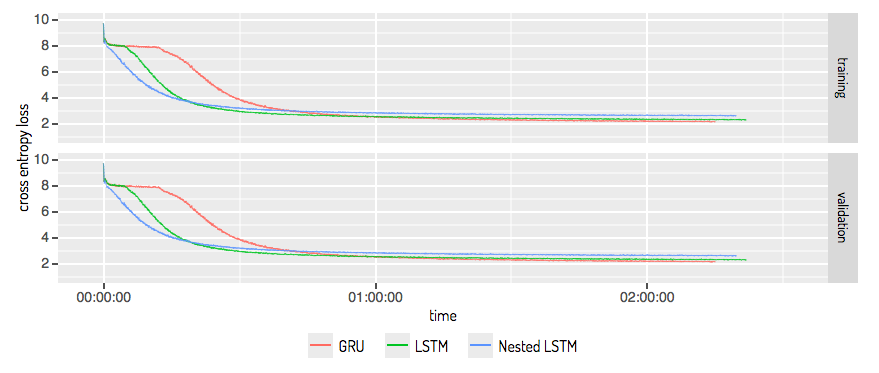Model training: Shows the training loss and validation loss for the GRU, LSTM, and Nested LSTM models when training on the autocomplete problem.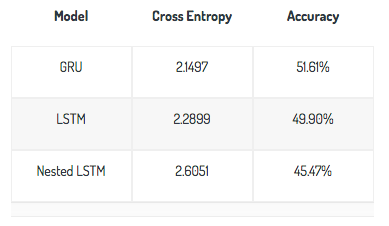As seen from the results the models are more or less equally fast. Surprisingly, the Nested LSTM is not better than the LSTM or GRU models. This somewhat contradicts the results found in the Nested LSTM paper, although they tested model on different problems and therefore, the results are not exactly comparable. Nevertheless, one would still expect the Nested LSTM model to perform better for this problem, where long-term memorization is important for the contextual understanding.

An unexpected result is that the Nested LSTM model initially converges much faster than the LSTM and GRU models. This, combined with the worse performance, indicates that the Nested LSTM optimizes forwards an unideal local minimum.

Conclusion

The Nested LSTM model did not provide any benefits over the LSTM or GRU models. This indicates, at least for the autocomplete example, that there isn't a connection between the number of internal memory states and the models ability to memorize and use that memory for contextual understanding.

Acknowledgments

Many thanks to the authors of the original Nested LSTM paper, Joel Ruben, Antony Moniz, and David Krueger. Even though our findings weren't the same, they have inspired much of this article and shown that something as used as the recurrent unit is still an open research area.

Topics:
ai ,neural network ,recurrent neural networks ,data science ,speech recognition ,machine translation

Comment (0)

Save
{{ articles.views | formatCount}} Views

Published at DZone with permission of

Opinions expressed by DZone contributors are their own.